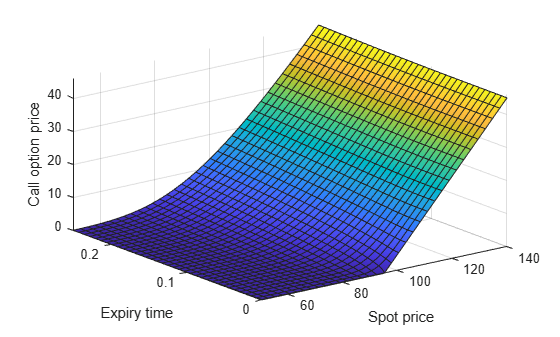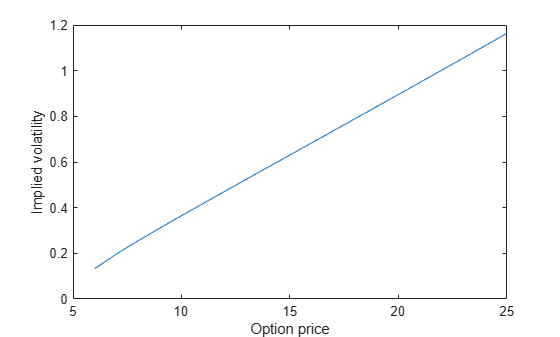# The Black–Scholes Formula for Call Option Price

This example shows how to calculate the call option price using the Black–Scholes formula. This example uses `vpasolve` to numerically solve the problems of finding the spot price and implied volatility from the Black–Scholes formula.

### Find Call Option Price

The Black–Scholes formula models the price of European call options . For a non-dividend-paying underlying stock, the parameters of the formula are defined as:

• $S$ is the current stock price or spot price.

• $K$ is the exercise or strike price.

• $\sigma$ is the standard deviation of continuously compounded annual returns of the stock, which is called volatility.

• $T$ is the time for the option to expire in years.

• $r$ is the annualized risk-free interest rate.

The price of a call option $C$ in terms of the Black–Scholes parameters is

$C=N\left({d}_{1}\right)×S-N\left({d}_{2}\right)×PV\left(K\right)$,

where:

• ${d}_{1}=\frac{1}{\sigma \sqrt{T}}\left[\mathrm{log}\left(\frac{S}{K}\right)+\left(r+\frac{{\sigma }^{2}}{2}\right)T\right]$

• ${d}_{2}={d}_{1}-\sigma \sqrt{T}$

• $PV\left(K\right)=K\mathrm{exp}\left(-rT\right)$

• $N\left(d\right)$ is the standard normal cumulative distribution function, $N\left(d\right)=\frac{1}{\sqrt{2\pi }}{\int }_{-\infty }^{d}\mathrm{exp}\left(-{t}^{2}/2\right)\phantom{\rule{0.2777777777777778em}{0ex}}dt$.

Find the price of a European stock option that expires in three months with an exercise price of \$95. Assume that the underlying stock pays no dividend, trades at \$100, and has a volatility of 50% per annum. The risk-free rate is 1% per annum.

Use `sym` to create symbolic numbers that represent the values of the Black–Scholes parameters.

```syms t d S = sym(100); % current stock price (spot price) K = sym(95); % exercise price (strike price) sigma = sym(0.50); % volatility of stock T = sym(3/12); % expiry time in years r = sym(0.01); % annualized risk-free interest rate```

Calculate the option price without approximation. Create a symbolic function `N(d)` that represents the standard normal cumulative distribution function.

```PV_K = K*exp(-r*T); d1 = (log(S/K) + (r + sigma^2/2)*T)/(sigma*sqrt(T)); d2 = d1 - sigma*sqrt(T); N(d) = int(exp(-((t)^2)/2),t,-Inf,d)*1/sqrt(2*sym(pi))```
```N(d) =  $\frac{\mathrm{erf}\left(\frac{\sqrt{2} d}{2}\right)}{2}+\frac{1}{2}$```
`Csym = N(d1)*S - N(d2)*PV_K`
```Csym =  $50 \mathrm{erf}\left(\frac{\sqrt{2} \left(4 \mathrm{log}\left(\frac{20}{19}\right)+\frac{27}{200}\right)}{2}\right)-95 {\mathrm{e}}^{-\frac{1}{400}} \left(\frac{\mathrm{erf}\left(\frac{\sqrt{2} \left(4 \mathrm{log}\left(\frac{20}{19}\right)-\frac{23}{200}\right)}{2}\right)}{2}+\frac{1}{2}\right)+50$```

To obtain the numeric result with variable precision, use `vpa`. By default, `vpa` returns a number with 32 significant digits.

`Cvpa = vpa(Csym)`
`Cvpa = $12.52792339252145394554497137187$`

To change the precision, use `digits`. The price of the option to 6 significant digits is \$12.5279.

```digits(6) Cvpa = vpa(Csym)```
`Cvpa = $12.5279$`

### Plot Call Option Price

Next, suppose that for the same stock option the time to expiry changes and the day-to-day stock price is unknown. Find the price of this call option for expiry time $T$ that varies from 0 to 0.25 years, and spot price $S$ that varies from \$50 to \$140. Use the values for exercise rate (`K`), volatility (`sigma`), and interest rate (`r`) from the previous example. In this case, use the time to expiry `T` and day-to-day stock price `S` as the variable quantities.

Define the symbolic expression `C` to represent the call option price with `T` and `S` as the unknown variables.

```syms T S PV_K = K*exp(-r*T); d1 = (log(S/K) + (r + sigma^2/2)*T)/(sigma*sqrt(T)); d2 = d1 - sigma*sqrt(T); Nd1 = int(exp(-((t)^2)/2),-Inf,d1)*1/sqrt(2*pi); Nd2 = int(exp(-((t)^2)/2),-Inf,d2)*1/sqrt(2*pi); C = Nd1*S - Nd2*PV_K;```

Plot the call option price as a function of spot price and expiry time.

```fsurf(C,[50 140 0 0.25]) xlabel('Spot price') ylabel('Expiry time') zlabel('Call option price')```Calculate the call option price with expiry time 0.1 years and spot price \$105. Use `subs` to substitute the values of `T` and `S` to the expression `C`. Return the price as a numeric result using `vpa`.

```Csym = subs(C,[T S],[0.1 105]); Cvpa = vpa(Csym)```
`Cvpa = $12.5868$`

### Find Spot Price

Consider the case where the option price is changing, and you want to know how this affects the underlying stock price. This is a problem of finding $S$ from the Black–Scholes formula given the known parameters $K$, $\sigma$, $T$, $r$, and $C$.

For example, after one month, the price of the same call option now trades at \$15.04 with expiry time of two months. Find the spot price of the underlying stock. Create a symbolic function `C(S)` that represents the Black–Scholes formula with the unknown parameter `S`.

```syms C(S) d1(S) d2(S) Nd1(S) Nd2(S) K = 95; % exercise price (strike price) sigma = 0.50; % volatility of stock T = 2/12; % expiry time in years r = 0.01; % annualized risk-free interest rate PV_K = K*exp(-r*T); d1(S) = (log(S/K) + (r + sigma^2/2)*T)/(sigma*sqrt(T)); d2(S) = d1 - sigma*sqrt(T); Nd1(S) = int(exp(-((t)^2)/2),-Inf,d1)*1/sqrt(2*pi); Nd2(S) = int(exp(-((t)^2)/2),-Inf,d2)*1/sqrt(2*pi); C(S) = Nd1*S - Nd2*PV_K;```

Use `vpasolve` to numerically solve for the spot price of the underlying stock. Search for solutions only in the positive numbers. The spot price of the underlying stock is \$106.162.

`S_Sol = vpasolve(C(S) == 15.04,S,[0 Inf])`
`S_Sol = $106.162$`

### Find Implied Volatility

Consider the case where the option price is changing and you want to know what is the implied volatility. This is a problem of finding the value of $\sigma$ from the Black–Scholes formula given the known parameters $S$, $K$, $T$, $r$, and $C$.

Consider the same stock option that expires in three months with an exercise price of \$95. Assume that the underlying stock trades at \$100, and the risk-free rate is 1% per annum. Find the implied volatility as a function of option price that ranges from \$6 to \$25. Create a vector for the range of the option price. Create a symbolic function `C(sigma)` that represents the Black–Scholes formula with the unknown parameter `sigma`. Use `vpasolve` to numerically solve for the implied volatility.

```syms C(sigma) d1(sigma) d2(sigma) Nd1(sigma) Nd2(sigma) S = 100; % current stock price (spot price) K = 95; % exercise price (strike price) T = 3/12; % expiry time in years r = 0.01; % annualized risk-free interest rate C_Range = 6:25; % range of option price sigma_Sol = zeros(size(C_Range)); PV_K = K*exp(-r*T); d1(sigma) = (log(S/K) + (r + sigma^2/2)*T)/(sigma*sqrt(T)); d2(sigma) = d1 - sigma*sqrt(T); Nd1(sigma) = int(exp(-((t)^2)/2),-Inf,d1)*1/sqrt(2*pi); Nd2(sigma) = int(exp(-((t)^2)/2),-Inf,d2)*1/sqrt(2*pi); C(sigma) = Nd1*S - Nd2*PV_K; for i = 1:length(C_Range) sigma_Sol(i) = vpasolve(C(sigma) == C_Range(i),sigma,[0 Inf]); end```

Plot the implied volatility as a function of the option price.

```plot(C_Range,sigma_Sol) xlabel('Option price') ylabel('Implied volatility')```